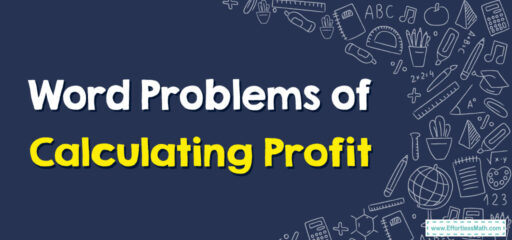# How to Solve Word Problems of Calculating Profit

Solving word problems involving calculating profit can be introduced to students by breaking them down into simple steps.## A Step-by-step Guide to Solving Word Problems of Calculating Profit

Here’s a step-by-step guide to help students solve word problems related to calculating profit:

### Step 1: Read the Problem Carefully

Read the word problem carefully to understand the situation and identify the relevant information. Pay attention to details about the cost, selling price, and any additional expenses or income mentioned.

### Step 2: Identify the Cost

Identify the cost of the item or service being sold. This is the amount of money spent or invested to produce or acquire the item. It might be mentioned explicitly in the problem or can be inferred from the context.

### Step 3: Identify the Selling Price

Determine the selling price, which is the amount at which the item is sold. This is usually provided in the problem or can be inferred from the information given.

### Step 4: Calculate the Profit

To find the profit, subtract the cost from the selling price. The formula to calculate profit is: Profit = Selling Price – Cost.

### Step 5: Analyze the Result

Evaluate the result to determine if there is a profit or loss. If the profit is positive, it means you made money. If the profit is negative, it means you experienced a loss.

### Step 6: Check for Additional Expenses or Income

Some word problems might include additional expenses or income that need to be taken into account. If there are any mentioned, add or subtract them from the profit calculation accordingly.

### Step 7: Practice with Examples

Provide practice word problems involving calculating profit for students to solve. Start with simpler examples and gradually increase the complexity as they gain confidence.

### Step 8: Review and Discuss

Review the solutions to the word problems as a class. Discuss the strategies used, highlight any common errors, and provide explanations for the correct answers.

By following this step-by-step guide, grade 4 students can develop their problem-solving skills and apply them to word problems involving calculating profit.

### What people say about "How to Solve Word Problems of Calculating Profit - Effortless Math: We Help Students Learn to LOVE Mathematics"?

No one replied yet.

X
30% OFF

Limited time only!

Save Over 30%

SAVE $5 It was$16.99 now it is \$11.99